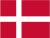### Dish Cloth 12

Another dish cloth made from yarn residues.

The pattern would perhaps have been better if knit in the same color alltogether.
Cast on 60 stitches and knit  6 rows. Start and end all rows with 6 knit. In between knit pattern 3 times in each row.
Pattern:
1.row: 1 k, 2 p, 2 k, 2 p, 3 k, 2 p, 2 k, 2 p
2.row: 2 p, 2 k, 7 p, 2 k, 2 p, 1 k
3.row: 1 p, 2 k, 2 p, 3 k, 1 p, 3 k, 2 p, 2 k
4.row: 2 k, 5 p, 1 k, 5 p, 2 k, 1 p
5.row: 1 k, 2 p, 3 k, 2 p, 1 k, 2 p, 3 k, 2 p
6.row: 5 p, 2 k, 1 p, 2 k, 5 p, 1 k
7.row: 1 p, 3 k, 2 p, 2 k, 1 p, 2 k, 2 p, 3 k
8.row: 3 p, 2 k, 2 p, 1 k, 2 p, 2 k, 4 p
9.row: 2 k, 2 p, 2 k, 2 p, 1 k, 2 p, 2 k, 2 p, 1 k
10.row:  knit as row 8
11.row:  knit as row 7
12.row:  knit as row  6
13.row:  knit as row  5
14.row:  knit as row  4
15.row:  knit as row  3
16.row: knit as row  2

 X X X X X 16 X X X X X X 15 X X X X X 14 X X X X X X X X 13 X X X X X 12 X X X X X X 11 X X X X X 10 X X X X X X X X 9 X X X X X 8 X X X X X X 7 X X X X X 6 X X X X X X X X 5 X X X X X 4 X X X X X X 3 X X X X X 2 X X X X X X X X 1

There are a lot of patterns on Pinterest. I made some mistakes in the beginning when reading the patterns. You have to remember that all uneven rows start in the right side of the diagram and X=purl and ▢= knit. All equal rows start in the  left side of the diagram and ▢= purl and X=knitDanish